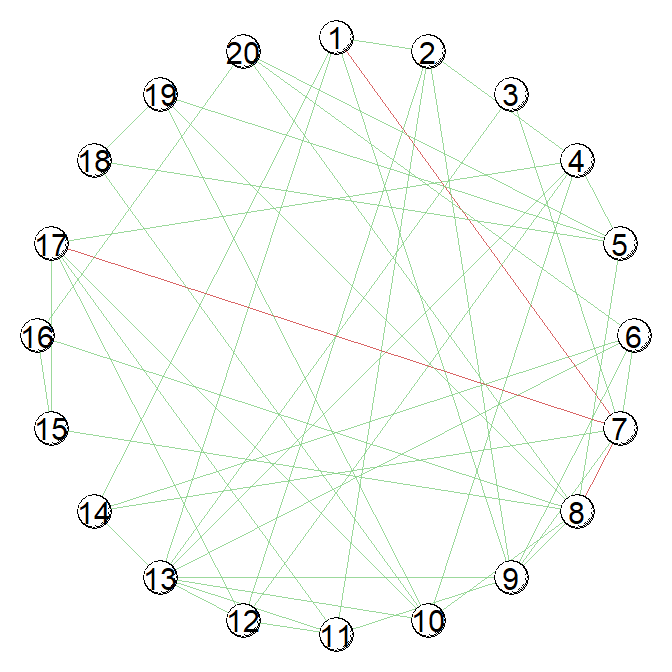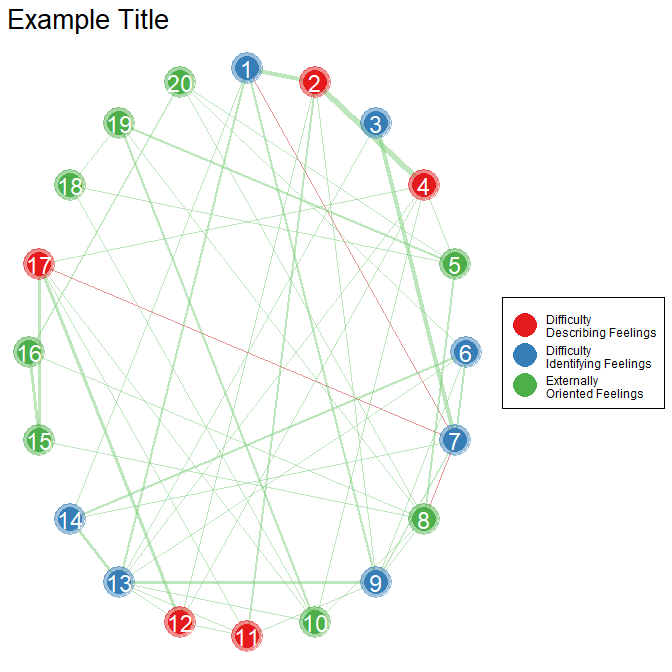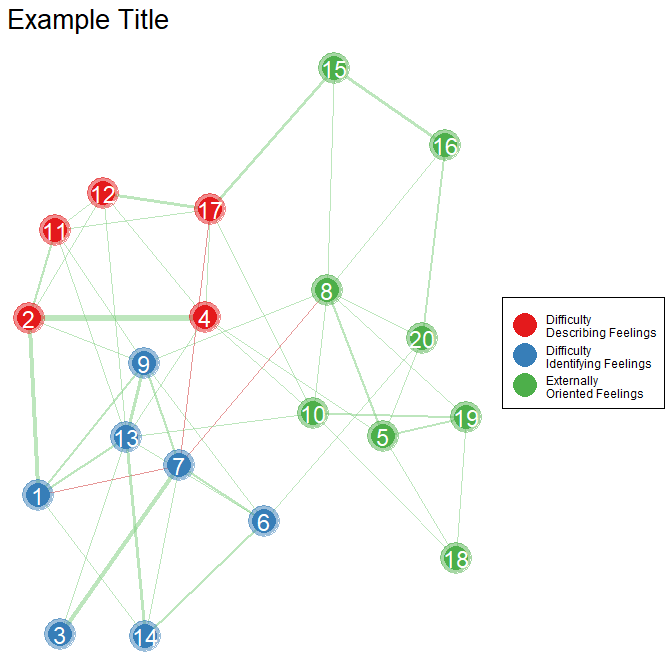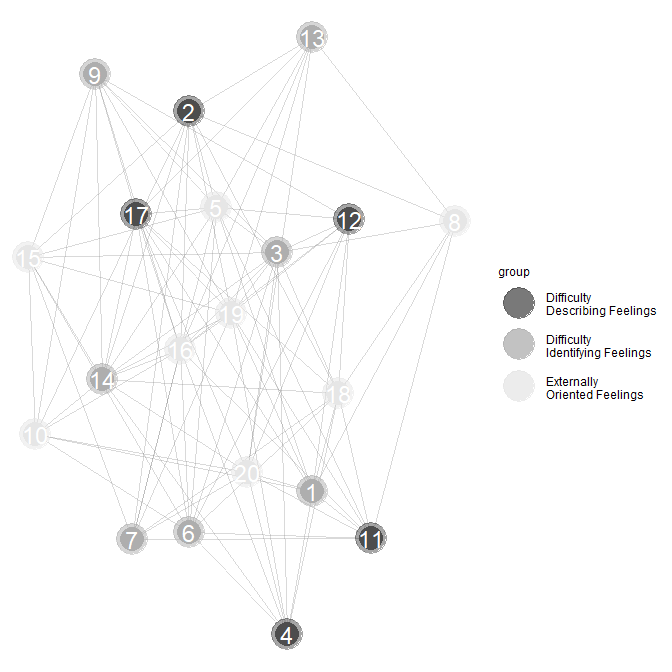# Background

A key aspect of psychological networks is visualizing the estimated structure (the “graph” in Gaussian graphical model). To this end, BGGM provides plotting capabilities for essentially all of the methods. For plotting the networks, BGGM is very elementary compared to the capabilities of the package qgraph. However, one advantage of BGGM is that the plots are ggplots which offers quite a bit of flexibility (especially for those familiar with ggplot2).

This vignette also provides the work flow for estimating networks with BGGM

## Estimate the Partial Correlations

dat <- subset(tas, select = - gender)

fit <- explore(dat,
prior_sd = 0.25,
iter = 5000)

## Select the Network

In this case, we are setting the threshold for the Bayes factor to 3. Hence, only Bayes factors exceeding that value will be included in network

sel <- select(fit, BF_cut = 3)

## Plot the Network

This will provide the simplest plots. There is one for non-zero relations and another for null effects.

plots <- plot(sel)

### Conditional Dependence Structure

The dependence structure refers to non-zero effects

plots$plt#### Customizing the Plot There are two ways to proceed for customizing the plot. The first is to take the plots object and do your thing in ggplot. Alternatively, there are some arguments that can be used with plot which should make a publication quality network. library(ggplot2) # change node names # add labels (e.g., group the items) # node names in white # node text size = 6 # change node size # increase edge width # transparency plots <- plot(sel, layout = "circle", node_labels_color = "white", node_groups = BGGM:::tas_labels, txt_size = 6, node_outer_size = 11, node_inner_size = 8, edge_multiplier = 5, alpha = 0.3) # remove legend name and set palette plots$plt +
scale_color_brewer(name = NULL,
palette = "Set1")  +
ggtitle("Example Title") +
# make title larger and add box around legend
theme(plot.title = element_text(size = 20),
legend.background = element_rect(color = "black"))
#> Scale for 'colour' is already present. Adding another scale for 'colour',
#> which will replace the existing scale.It is also possible to change the layout. In my experience, however, using plotting algorithms should be done with caution. This is because it can lead to overinterpreting the placement of nodes, when really this is mere speculation. To avoid this I prefer the circle layout.

Nonetheless, here is an example of a different layout (many are available)

# add different layout
plots <- plot(sel, "fruchtermanreingold",
node_labels_color = "white",
node_groups = BGGM:::tas_labels,
txt_size = 6, node_outer_size = 11,
node_inner_size = 8,
edge_multiplier = 5,
alpha = 0.3)

# further customize
plots$plt + # remove legend name and set palette scale_color_brewer(name = NULL, palette = "Set1") + # add title ggtitle("Example Title") + # make title larger and add box around legend theme(plot.title = element_text(size = 20), legend.background = element_rect(color = "black")) #> Scale for 'colour' is already present. Adding another scale for 'colour', #> which will replace the existing scale.### Conditional Independence Structure The conditional independence structure refers to null effects. plots$plt_null